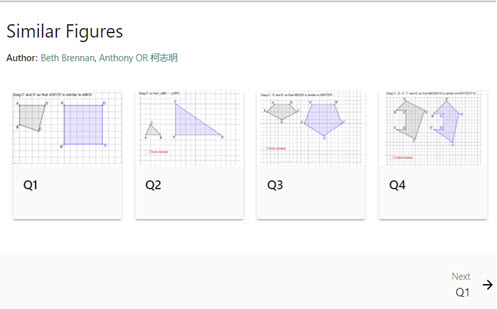Explore

# Similar figures

These four questions challenge students to create similar figures using dynamic geometry.

Year level(s) Year 9
Audience Student
Teaching strategies and pedagogical approaches Feedback
Keywords Transformation, Ratio, Triangle, Angle, Proof, Congruent, Similar, Scale factor

## Curriculum alignment

Curriculum connections Critical and creative thinking, Numeracy
Strand and focus Space
Topics Angles and geometric reasoning
AC: Mathematics (V9.0) content descriptions
AC9M9M03

Solve spatial problems, applying angle properties, scale, similarity, Pythagoras' theorem and trigonometry in right-angled triangles

Numeracy progression Understanding geometric properties (P7)
Proportional thinking (P7)
Understanding units of measurement (P10)